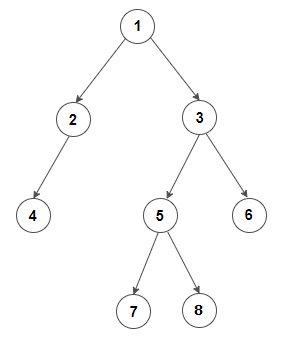# How To Find preorder trave?rsal of a binary tree from its inorder and postorder sequence?

Write an efficient algorithm to find preorder traversal of a binary tree from its inorder and postorder sequence without constructing the tree.
For example, consider below tree:Input:
Inorder Traversal:   { 4, 2, 1, 7, 5, 8, 3, 6 }
Postorder Traversal: { 4, 2, 7, 8, 5, 6, 3, 1 }

Output:
Preorder Traversal is: { 1, 2, 4, 3, 5, 7, 8, 6 }

We start with the root node whose value would be the last item in the postorder sequence. The idea is to find boundaries of the left and right subtree of root node in the given inorder sequence. To find the boundaries of left and right subtree, we search for index of the root node in inorder sequence. Now all keys before the root node in inorder sequence becomes part of the left subtree and all keys after the root node becomes part of the right subtree. If we repeat this recursively for all nodes of the tree, we will end up doing a preorder traversal of the tree. This is demonstrated below in C++ –

Output:

Preorder Traversal is: 1 2 4 3 5 7 8 6

The time complexity of above solution is O(n) and requires O(n) extra space for std::unordered_mapstd::stack, and recursive call stack.